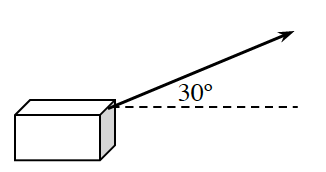### Home > PC > Chapter 10 > Lesson 10.1.4 > Problem10-55

10-55.To slide a crate across a floor, a rope is attached and pulled with a constant force of $50$ pounds at an angle of $30^\circ$. How much work is done if the box is dragged $10$ feet?

$\text{work} = \left(\text{force}\right) · \left(\text{distance}\right)$

Which component of the given vector is actually moving the box forward?

Determine the magnitude of the horizontal component of the given vector.

Since this is a $30^\circ-60^\circ-90^\circ$ triangle, $y = 25$ and $x = 25\sqrt{3}.$

$\text{work} = \left(25\sqrt{3} \text{ pounds}\right)\left(10 \text{ feet}\right)$